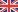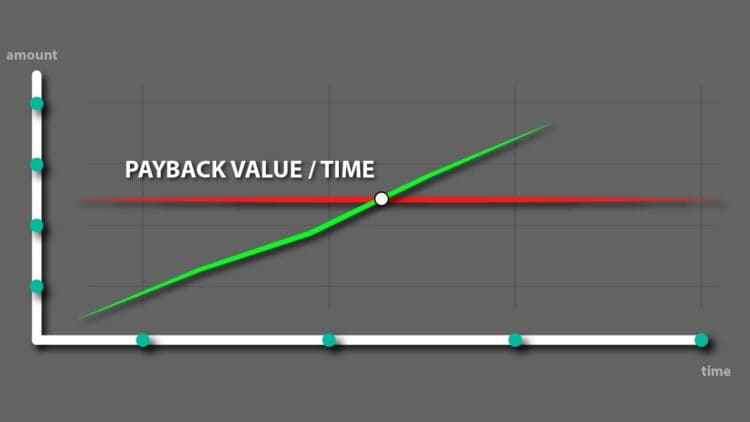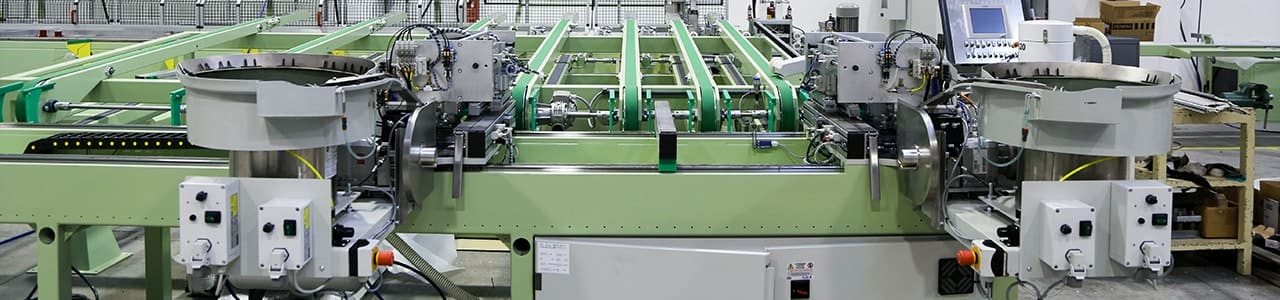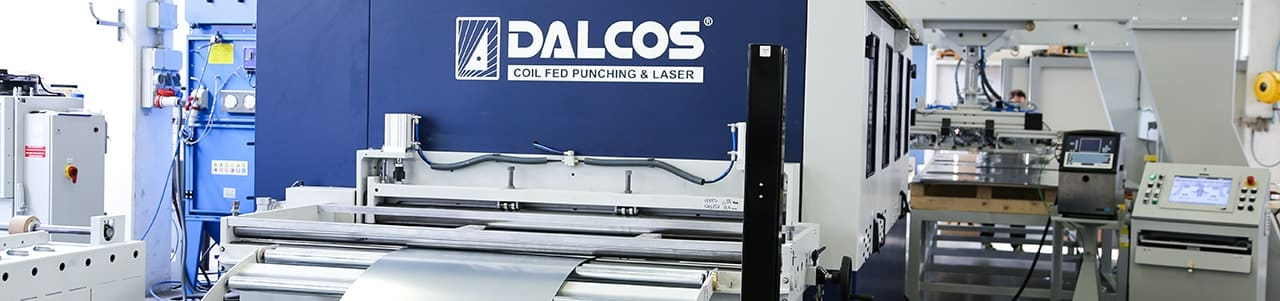Change language

# Sheet metal working machines: how to calculate the investment payback period

September 2017When investing in a new machine, it is interesting to calculate how much time it will take for the investment to be paid back.

The method I suggest is very simple, comparing the value of the investment with the savings (corresponding to the cash flow) generated by the new production line.

## New Machine cycle time, Cost per hour, Efficiency

I have covered in the previous article how to calculate the manufacturing cost of a sheet metal product: we will use the results of the same calculations to identify the production cost per part of the new production line.

To calculate the hourly cost of the machine, we start from the formula taken from my article “How to calculate the hourly cost of a sheet metal working machine:

#### (Total Hourly Cost) = (Investment HC) + (Electricity HC) + (Labor HC) + (Maintenance HC) + (Consumables HC) + (Occupied area HC)

This formula needs to be modified, removing the Investment Hourly Cost factor to find the Operating Cost of the line:

#### (Operating Cost) = (Electricity HC) + (Labor HC) + (Maintenance HC) + (Consumables HC) + (Occupied area HC)

Infact, the Investment Hourly Cost requires to estimate the payback period, which is what we need to calculate now. These costs are the mere operating costs of the line.

Using the following formula, we can calculate the cost of the product in the new machine.

#### (Product cost in new machine) = (Raw materials cost) + (Operating Cost) * (Cycle time per one piece) / (Efficiency)

The details on how to get to the formula can be found here.

For example, a product with: raw material cost 1,91 Euro, Cycle time 12 seconds, Operating Cost 21,8 Euro per hour, Efficiency 80,5% gives a Product Cost of 2 Euros.

## Comparison of production cost with present product cost

If the product output of the machine is now purchased (make or buy analysis), we can calculate the earnings generated by the new investment per piece with this formula:

#### (Earning per piece) = (Purchase price of the product) – (Product cost in new machine)

In case the product is manufactured with a different manufacturing cycle, we can calculate the earnings in this way:

#### (Earning per piece) = (Production cost in old machine) – (Production cost in new machine)

Total production cost in the old machine can be either calculated, derived from internal databases or estimated.

For example, if the product in the form it comes out of the machine was purchased at a cost of 5 Euro, the earning per piece would be 3 Euro.

## Calculating the pay back of the investment

It is easy to calculate the number of parts N that pay back the investment.

#### N = (Total cost of investment) / (Earning per piece)

For example, for an investment of 300000 Euros we obtain:

#### N = 500.000 / 3 =166.000 parts

And from this calculation, we can calculate how much time is required for the payback:

#### (Payback time) = N * (cycle time per one piece) / Efficiency

In the previous example:

N = 166.000 * 12 / 0,805 / 3600 = 687 Hours

## Important notes

In case there are multiple parts in the production, it is necessary to estimate an average cycle time based on the produced quantities (weighted average).

Normally, 20% of the codes that are run into a machine make up the 80% of the production time (80/20 rule). If we focus on these items, we will have anyway a good estimate.

## Examples of simplified payback calculations

Quite often, more simplified methods are used for the calculation of the payback. These are simply taking into account just a part of the factors of the previous formula: here we’ll see some examples.

### Comparing the value of the investment with the savings generated in the raw materialIn one previous article I analyzed how much material a sheet metal manufacturing company is saving by moving just seven articles from sheet to coil fed fabrication. It turned out that almost 16% of the material is saved by the coil fed processing.

This results in a saving of 95.200 Euro per year. Now, this figure can be compared to the value of the investment, for example 500.000 Euro; the payback time just for the raw material saving is 5,25 years.

Another example: soon we’ll publicate a new article on the raw material saving in the production of rolling shutters. Modern manufacturing systems utilize a coil-to-window process that allow for savings between 10 and 15% of the raw materials.

Just analyzing the saving in the usage of aluminum and polyurethane, companies using this method of production generate savings between 100.000 and 220.000 Euro per year.

Again, it is enough to divide the value of the investment by the generated saving to get a conservative value of the payback time for the investment.

Note that, since this method does not sum up the other factors of the formula, it is generally very conservative and the payback time turns out to be shorter. For example, in the coil to window automated systems, both the increased production speed and the reduced labor costs generate additional savings that make the payback time extremely short.

### Comparing the value of the investment with the savings generated by the increased speedThe higher production speed and reduced operating costs generate savings that can be used to compare the performance of two machines and their payback time.

In one previous article, I showed how the company Elleci calculated the processing time in two different machines. The existing one produced the parts in 3900 hours (almost two shifts), the coil fed punch/laser combo in less than 1700 hours (less than one shift).

Elleci estimated an operation cost in 23 Euro per hour (based only on manpower and electricity) for both machines.

The difference in the cost of the processing time results in 51.100 Euro per year.

Since the coil fed punch/laser machine has extra capacity due, they operate it in two shifts and calculate a total saving of 102.200 Euro.

Note: this calculation method is also extremely conservative. In fact, it does not take into account that, with the previous technology (sheet fed), the company should have purchased a second sheet fed machine to manufacture the same amount of products.

### Comparing the value of the investment with the savings from automated processes

Another simplified way to estimate the payback of the investment is the saving generated by the increased automation and, consequently, by the reduced labor cost.

We see this approach especially in countries where cost per operator is higher, or where it is difficult to find operators for the specific job.

This calculation compares the number of operators that are required to complete the production without the automation, with the number of operators required to operate the automated line.

Again, it is enough to divide the value of the investment by the savings generated by the reduced labor to estimate the payback time.

As the previous ones, this methodology is approximate: automated processes usually generate an increased productivity and saving in raw materials as well.

## Conclusions

When investing in a new machine, production managers are more and more required to do accurate calculations in order to calculate the Return on the Investment.

Calculating the payback time with the suggested formula – or one of the simplified methodologies – gives an immediate feeling on the quality of the investment and the cash flow it generates.

This website uses cookies to improve user experience. By clicking the enable button you get the best experience on the website with full contents, consenting to all cookies in accordance with our  Cookie policy.